• +91 9971497814
• info@interviewmaterial.com

# Introduction to Trigonometry Ex-8.3 Interview Questions Answers

### Related Subjects

Question 1 : Evaluate :

(i) sin 18°/cos 72°                (ii) tan 26°/cot 64°

(iii)  cos48° – sin 42            (iv)  cosec31° – sec 59°

Solution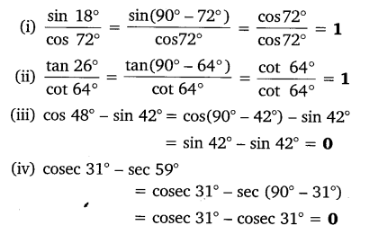Question 2 :

Show that:

(i) tan 48° tan 23° tan 42° tan 67° = 1

(ii) cos 38° cos 52° – sin 38° sin 52° = 0

Answer 2 :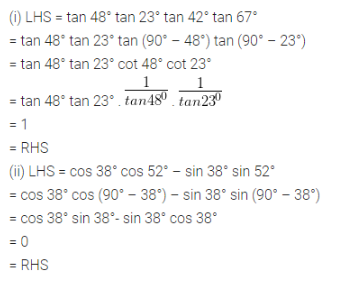Question 3 :

If tan 2A = cot (A –18°), where 2A is an acute angle, find the value of A.

Answer 3 :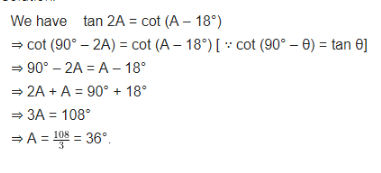Question 4 :

If tan A = cot B,prove that A + B = 90°.

Answer 4 :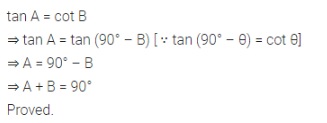Question 5 :

If sec 4A = cosec (A– 20°), where 4A is an acute angle, find the value of A.

Answer 5 :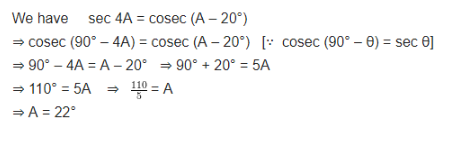Question 6 :

If A, B and C areinterior angles of a triangle ABC, then show that

sin(B+C/2) = cos A/2

We know that, for a given triangle, sum of allthe interior angles of a triangle is equal to 180°

A + B + C = 180° ….(1)

To find the value of (B+ C)/2, simplify theequation (1)

B + C = 180° – A

(B+C)/2 = (180°-A)/2

(B+C)/2 = (90°-A/2)

Now, multiply both sides by sin functions, weget

sin (B+C)/2 = sin (90°-A/2)

Since sin (90°-A/2) = = cos A/2, the aboveequation is equal to

sin (B+C)/2 = cos A/2

Hence proved.

Question 7 :

Express sin 67° +cos 75° in terms of trigonometric ratios of angles between 0° and 45°.

Given:

sin 67° + cos 75°

In term of sin as cos function and cos as sinfunction, it can be written as follows

sin 67° = sin (90° – 23°)

cos 75° = cos (90° – 15°)

= sin (90° – 23°) + cos (90° – 15°)

Now, simplify the above equation

= cos 23° + sin 15°

Therefore, sin 67° + cos 75° is also expressedas cos 23° + sin 15°

Todays Deals### Introduction to Trigonometry Ex-8.3 Contributorskrishan

Name:
Email:

# Latest News# 9000 interview questions in different categories